# 3.2 First plots

The core graph-plotting command of Pyxplot is the plot command. The following simple example plots the trigonometric function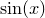:

plot sin(x)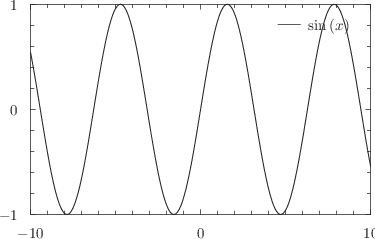This is one of a large number of standard mathematical functions which are built into Pyxplot; a complete alphabetical list of them can be found in Chapter 12.

It is also possible to plot data stored in files. The following would plot data from a file data.dat, taking the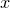-coordinate of each point from the first column of the data file, and the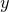-coordinate from the second. The data file is assumed to be in plain text format1, with columns separated by whitespace and/or commas2:

plot 'data.dat'


Several items can be plotted on the same graph by separating them by commas, as in

plot 'data.dat', sin(x), cos(x)


and it is possible to define one’s own variables and functions, and then plot them, as in the example

a = 0.02
b = -1
c = 5
f(x) = a*(x**3) + b*x + c
plot f(x)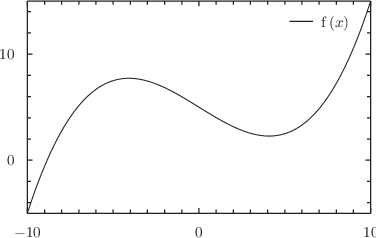Pyxplot supports almost all of the same mathematical operators as the C programming language; a complete list of them can be found in Table 3.1. If you have experience of similar tricks in C, it is quite possible to write the following expressions in Pyxplot (but don’t worry if this is a little over your head):

pyxplot> print (a=3)+(b=2)
5
pyxplot> print a0?"yes":"no"
yes
pyxplot> print "%s  %s"%(++a,b++)
4  2
pyxplot> print (a+=10 , b+=10 , a+b)
27

In the final example, the comma operator is used as in C, to return only the value of the final comma-separated expression.

 Symbol Description Operator Associativity ** Algebraic exponentiation right-to-left - Unary minus sign right-to-left – Unary decrement right-to-left + Unary plus sign right-to-left ++ Unary increment right-to-left not  ! Logical not right-to-leftUnary one’s complement right-to-left * Algebraic multiplication left-to-right / Algebraic division left-to-right % Modulo operator left-to-right + Algebraic sum left-to-right - Algebraic subtraction left-to-right << Left binary shift left-to-right >> Right binary shift left-to-right < Magnitude comparison right-to-left > Magnitude comparison right-to-left <= Magnitude comparison right-to-left >= Magnitude comparison right-to-left ==  <> Equality comparison right-to-left != Equality comparison right-to-left & Binary and left-to-rightBinary exclusive or left-to-right | Binary or left-to-right and  && Logical and left-to-right or  || Logical or left-to-right ?: Ternary conditional right-to-left = Assignment operator right-to-left += -= *= Assignment operators right-to-left /= %= &== |= <<= >>= , Comma separator left-to-right
Table 3.1: A list of mathematical operators which Pyxplot recognises, in order of descending precedence. Items separated by horizontal rules are of differing precedence; those not separated by horizontal rules are of equal precedence. The third column indicates whether strings of operators are evaluated from left to right, or from right to left. For example, the expression x**y**z is evaluated as (x**(y**z)).

Footnotes

1. If the filename of a data file ends with a .gz suffix, it is assuming to be gzipped plaintext, and is decoded accordingly. Other formats of data file can be opened with the use of input filters; see Section 5.1.
2. This format is compatible with the Comma Separated Values (CSV) format produced by many applications such as Microsoft Excel.